Ch 4. The Mean Value Theorem Multimedia Engineering Math Maximum & Minimum Rolle'sTheorem Mean ValueTheorem MonotonicFunctions First DerivativeTest Concavity &Inflection SecondDerivative Test
 Chapter 1. Limits 2. Derivatives I 3. Derivatives II 4. Mean Value 5. Curve Sketching 6. Integrals 7. Inverse Functions 8. Integration Tech. 9. Integrate App. 10. Parametric Eqs. 11. Polar Coord. 12. Series Appendix Basic Math Units Search eBooks Dynamics Fluids Math Mechanics Statics Thermodynamics Author(s): Hengzhong Wen Chean Chin Ngo Meirong Huang Kurt Gramoll ©Kurt GramollMATHEMATICS - THEORY In engineering, there are many optimization problems which can be reduced to finding the maximum or minimum values of a given function. In this section, the basic concept and theorem related to maximum and minimum are discussed. Maximum Value and Minimum Value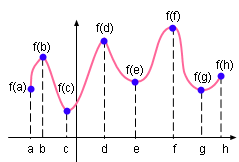Example Description Diagram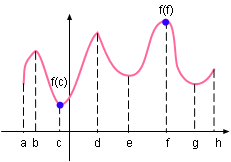Maximum and Minimum Value Maximum value is the absolute maximum value of the function in its domain. In mathematics it is defined as following: A function f has an absolute maximum at point x0 if f(x0) ≥ f(x) for all x in its domain D. The number f(x0) is called the maximum value of f on its domain. For example, the maximum value of the function plotted on the left is f(f) between a and h. Similarly, minimum value is the absolute minimum value of the function in its domain. In mathematics it is defined as following: Function f has an absolute minimum at point x0 if f(x0) ≤ f(x) for all x in its domain D. The number f(x0) is called the minimum value of f on its domain. The minimum value for the function shown on the left is f(c) in [a, h]. The maximum and minimum values of the function are called the extreme values of the function. In the function show on the left, f(c) and f(f) are the extreme values. Local Maximum and Local Minimum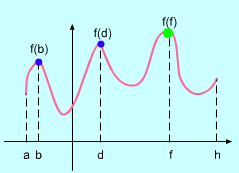Maximum Value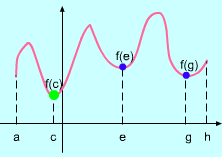Minimum Value The local maximum is defined in mathematics as: A function f has a local maximum at x0 if there is an open interval u that contains x0 such that f(x0) ≥ f(x) for all x in this interval u. Local maximum is also called relative maximum. In Example Description Diagram, f(b), f(d) and f(f) are the local maximum. Notice that f(f) is also absolute maximum and extreme value of the function. Similarly, local minimum is defined as, Function f has local minimum at x0 if there is an open interval u that contains x0 such that f(x0) ≤ f(x) for all x in this interval u. Local minimum is also called relative minimum. In the previous example, f(c), f(e) and f(g) are the local minimum. Notice that f(c) is also absolute minimum and extreme value of the function. Extreme Values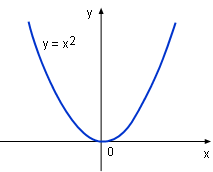Function y = x2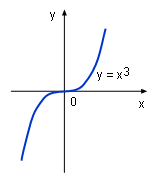Function y = x3 Some functions have extreme value such as function y = x2. Since in (-∞, +∞ ), f(x) ≥ f(0). Therefore, f(0) = 0 is the absolute and local minimum value of this function. It is also the extreme value. However, some functions do not have extreme value such as function y = x3. In the function's domain (-∞, +∞ ), it does not have an absolute maximum or an absolute minimum value. In reality, it has no local maximum and local minimum too. and thus function y = x3 does not have extreme value. The Extreme Value Theorem has been introduced in the Continuity sectiion. It gives the conditions under which a function has an extreme value.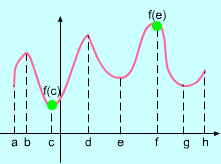Extreme Value Fermat's Theorem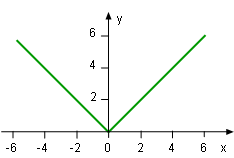Function y = (x2)0.5 The Fermat's Theorem can be used to find the location of an extreme value. The theorem states, If a function has a local extreme which is the maximum or minimum at x0, and if df(x0)/dx exist, then df(x0)/dx = 0. However, using Fermat's Theorem does not guarantee to find all extreme value. For example, although the absolute and local minimum value is 0 for function y = (x2)0.5, but this value cannot be found by setting df(x0)/dx = 0. The reason is df(0)/dx does not exist which has been shown in the Differentiability section. Fermat's Theorem suggest that looking for extreme values at point x0 where df(x0)/dx = 0 or df(x0)/dx does not exist. Such points are given a special name - critical point. Critical Point A critical point is the point where the derivative of the function is 0 or does not exist. The method of calculating the critical point can be understood by finding the critical point of function f(x) = 6x1/3 - 5x. Calculating the derivative of f(x) gives      df(x)/dx = d( 6x1/3)/dx - d(5x)                  = 2x-2/3 - 5 When df(x)/dx = 0      2x-2/3 - 5 = 0 Therefore, x = 0.34 When df(x)/dx does not exist,      x-2/3 = 0. So x = 0 Thus, the critical point is at x = 0.34 and x = 0. In terms of critical points, Fermat's Theorem can be rephrased as: If a function has a local extreme at x0, then x0 is a critical point of the function. Method of Finding Absolute Maximum and Minimum In order to find the absolute maximum and minimum of a continuous function in its domain [a, b], the following steps need to be taken: Find the value of f(a) and f(b). Find the critical points of the function and calculate the value at critical points. Compare the result of the above steps. The absolute maximum value is the largest one and the absolute minimum is the smallest one.